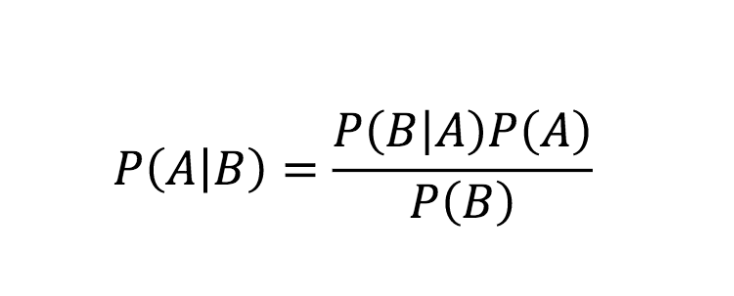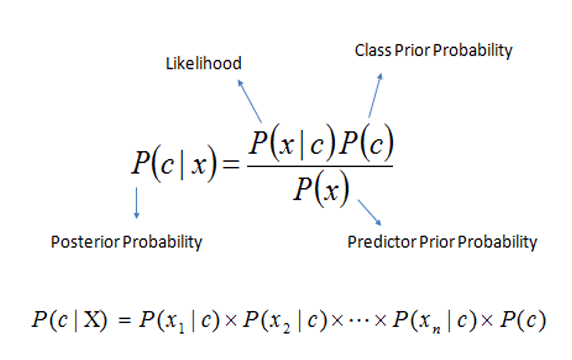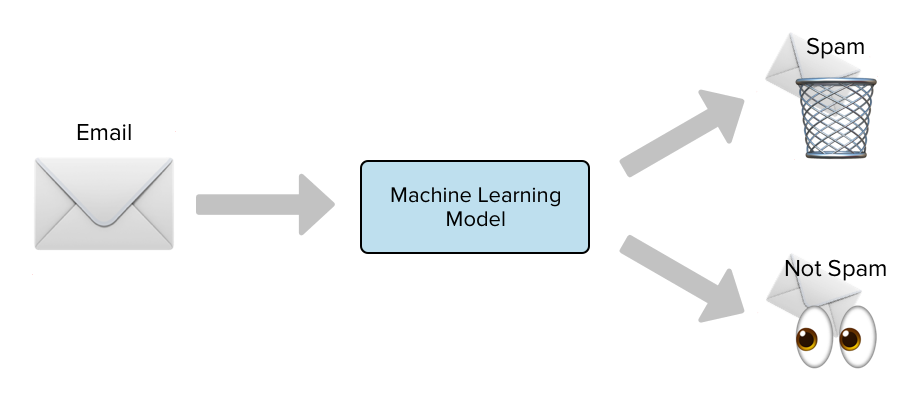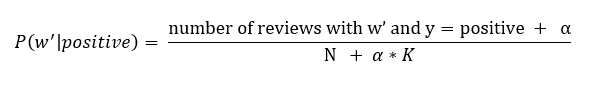# Laplace Smoothing

## Introduction

The Naive Bayes algorithm is a subset of Supervised Learning Algorithms. It is established from the Bayes theorem and used to solve classification problems using a probabilistic method. Some famous examples of the Naive Bayes algorithm are Spam filtration, text classification, a recommendation system, and sentimental analysis.

## Bayes Theorem

Bayes Theorem calculates the probability of an event occurring based on the previous occurring outcome. The mathematical representation of the Theorem is:Where,

P(c/x) is the posterior probability, i.e., the possibility of event A occurring, given event B has already occurred.

P(x/c) is the likelihood probability, i.e., the chance of event B occurring, given event A has already happened.

P(c) is the prior probability that is the probability of event A happening

P(x) is the marginal probability that is the probability of event B occurring

The Bayes Theorem equation can also be rewritten as:## Assumptions of Naive Bayes

The two main assumptions are that the vectors are independent, and there is no correlation. Another assumption is that all the features play an equal role, i.e., all the vectors are not correlated with each other and do not cause redundancy. To get an in-depth understanding of Naive Bayes, check out this article.

## Laplace Smoothing

While testing the model, there could be a situation where the model has faced a new query point, a data point for which it is not trained. According to the Naive Bayes formula, the posterior probability would become zero for that particular data point. Hence the resulting possibility after multiplication will also be zero.

For example, we have built a word finder model, and we have to find the word 'sport' in a sentence, but the model is not trained for that word. Then the probability of sport, i.e., P(word/sport), is zero, and after we multiply the possibilities, the product will be zero.

Laplace smoothing was introduced to overcome this error.

## How does Laplace Smoothing work?

Let us understand this with the help of the spam classifier example.Suppose the word gift was only included in the spam emails during the training period. Therefore, you assume that gift is a spam word and conclude that

i.e., none of the non-spam emails would have the word gift in them.

The probability of this event occurring is low but not zero. Later as we have to multiply all the probabilities, the final result will be zero, and even the non-spam mail will be concluded as spam.

That is why we need Laplace smoothing; it ensures that the posterior probability is never zero. It increases the zero probability values to small positive values and simultaneously reduces other matters so that the final sum remains to be one.

We modify the posterior probability formula in the following way:In the formula mentioned above,

Alpha is the number of smoothing parameters

K represents the number of parameters.

N represents the number of reviews considering y is positive.

It ensures no case of zero prior probability and appropriately executes the classification.

Since the mathematical terms are changed to give a better classification, the actual probabilities of the event are changed. Also, to increase the value of the zero probability data point, the rest of the data point's possibilities are reduced to maintain the law of probability.

1. What is meant by pseudo count?
Ans. The term pseudo count means to add some value in the observed cases to ensure there is no zero probability problem.

2. Why is Laplace smoothing important in naive Bayes?
Ans. Laplace smoothing is crucial because it classifies the terms into more possible classes than only two.

3. Why do we need smoothing?
Ans. Applying Laplace smoothing gives the classifier more options to classify the probabilities over more diverse events.

## Key Takeaways

Laplace Smoothing is a technique that removes the problem of zero probability in the Naive Bayes Algorithm. This article studied Laplace smoothing, how it works, and its advantages and disadvantages. To build a career in Data Science? Check out our industry-oriented machine learning course curated by our faculty from Stanford University and Industry experts.